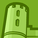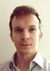# New to Qlik Sense

If you’re new to Qlik Sense, start with this Discussion Board and get up-to-speed quickly.

Announcements
Qlik Insider: SaaS capabilities for Data Integration and Analytics, May 25th! Register Now
cancel
Showing results for
Did you mean:Contributor II

## Alternative State on Y-Axis in Distribution Chart

Hello,

I am trying to have 2 alternative states to be plotted similarly to how a bar chart does with 2 calculated measures using the set expressions i.e. Avg({[Sample-A]}[x]). What i am looking to do is have Sample-A & Sample-B on the Y-axis (Chart rotated) if that is at all possible?

My thinking would be a Dimension simply with "A" and "B" for example if the field is in either of the states but i am not sure how to proceed.

Thanks

1 Solution

Accepted SolutionsContributor III

Hi,

You could create a dummy dimension 'sample' in your data load with values 'A' and 'B' and use this as the dimension for the distribution chart.

Then use an if statement such as this one in the measure:

=if(only(sample) = 'A', Avg({[Sample-A]} x), Avg({[Sample-B]} x))

Does that help?

Ben

2 RepliesContributor III

Hi,

You could create a dummy dimension 'sample' in your data load with values 'A' and 'B' and use this as the dimension for the distribution chart.

Then use an if statement such as this one in the measure:

=if(only(sample) = 'A', Avg({[Sample-A]} x), Avg({[Sample-B]} x))

Does that help?

BenContributor II
Author

That works great thank you!

I am hoping to reuse it in a T-test as well but have not tried it yet.